• 方法：程序采用巴特沃斯低通滤波和椭圆低通滤波，其中，备注对涉及到的函数进行通俗的解释，并画出了滤波器的频率响应特性和时域、频域滤波结果。其中还对两个滤波函数(fftfilt, filtfilt)得结果进行对比，显示了...
• 采用自带的buttord 和butter函数实现对信号的低通滤波
• 精选文档 DSP设计滤波器报告 一低通滤波器的设计 一实验目的掌握 IIR 数字低通滤波器的设计方法 二实验原理 1滤波器的分类 滤波器分两大类经典滤波器和现代滤波器 经典滤波器是假定输入信号 x( n) 的有用成分和...
• 低通滤波器MATLAB程序 ，值得借鉴。附带快速傅里叶变换的子程序
• 基于 matlab低通滤波器 摘要:调用 MATLAB 信号处理工具箱滤波通过观察滤波器输入输出信号的时域波形及其频谱建立数字滤波的概 念应用最广泛的是双线性变换法基本设计过程是先将给定的数字滤波器的指标转换成...
• 任务：设计一个截止频率为15.9kHz的RC低通滤波器，用MATLAB仿真软件仿真输入输出信号的时域波形、频域波形、自相关函数、功率谱密度等，然后在面包板上制作该滤波器，最后测试滤波器输入、输出信号的时域波形、频域...
• 本程序是用matlab实现的FIR低通滤波器，通过加载一段音频文件，进行滤波处理，最后又回放滤波器后的音频文件…… 可以通过听觉上区分滤波前后的差别，更好的方式当然是通过观察滤波前后的频谱来进行验证……
• 基于matlab 的巴特沃斯低通滤波器实现 一 课程设计的目的 运用MATLAB实现巴特沃斯低通滤波器的设计以及相应结果的显示另外还对多种低通滤波窗口进行了比较 二课程设计的基本要求 1熟悉和掌握MATLAB 的基本应用技巧...
• matlab开发-低通滤波器设计。基于约束二次规划（QP）的低通FIR数字微分器（LPFIRDD）设计。
• 要使用过滤器： 1）在变量img创建一张图片2) 调用函数 filter 来创建与图像 'img' 大小相同的过滤器 在滤波器代码指定截止频率 d0 的值。
• 1、利用matlab求矩形窗的DFT2、利用DFT分别计算已知两个序列的圆周卷积和线性卷积3、用汉宁窗设计一个数字带通滤波器4、设计巴特沃斯数字低通滤波器
• 本资源为一个matlab源代码和一个纯净的音乐信号，通过对音乐信号加高频余弦噪声，得到掺杂高频余弦噪声的音乐信号；后通过设计巴特沃斯低通滤波器以及频域分析，实现高频噪声的去除，重新得到纯净的音乐信号。
• 理想低通滤波器 (ILPF)：简单地切断所有距离变换原点指定距离
• 采用matlab实现巴特沃斯低通滤波，对信号Mix_Signal_1 和 Mix_Signal_2 分别作巴特沃斯低通滤波
• 低通滤波器概念有许多不同的形式，其中包括电子线路（如音频设备使用的hiss 滤波器）、平滑数据的数字算法、音障（acousc barriers）、图像模糊处理等等，这两个工具都通过剔除短期波动、保留长期发展趋势提供了...
• 此函数计算低通滤波器（N 阶）级联设计的截面排序和增益分布，如 R. Schaumann, H. 所述。 Xiao，ME Van Valkenburg，《模拟滤波器设计》，牛津大学出版社，2011年，第246-257页。
• matlab中如何去除高振幅的...从图第三个子图可以看到 ，基波信号都在0.05Hz以下，所以用一阶低通滤波器就可以了。第二步，使用 一阶低通滤波器，然后plot。下面分别为 截至频率，0.1，0.2Hz的滤波器， 源码放在下...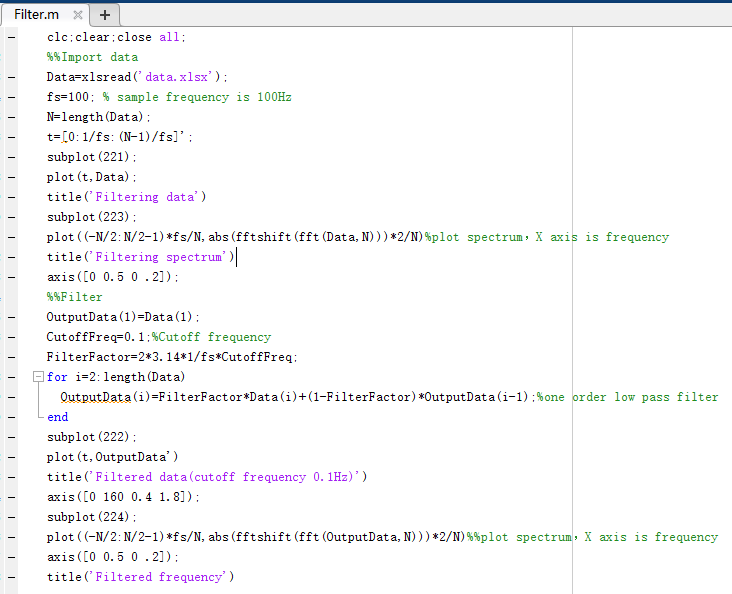matlab中如何去除高振幅的干扰信号？​www.zhihu.com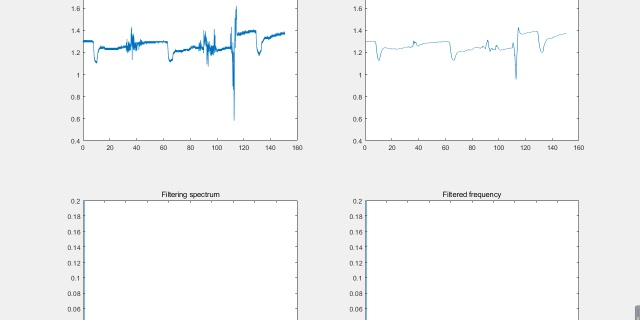题主没有给出采样频率，或采样时间，数据中没有，我们就假定采样时间为 0.01s。
第一步， 电压信号读入，然后傅里叶分析，得到原信号频谱图。从图中第三个子图可以看到 ，基波信号都在0.05Hz以下，所以用一阶低通滤波器就可以了。
第二步，使用 一阶低通滤波器，然后plot。
下面分别为 截至频率，0.1，0.2Hz的滤波器， 源码放在下面了，可以尝试调一调。

这里的一个基本概念就是 ：
一阶低通滤波，又叫一阶惯性滤波，或一阶低通滤波。是使用软件编程实现普通硬件RC低通滤波器的功能。一阶低通滤波法采用本次采样值与上次滤波输出值进行加权，得到有效滤波值，使得输出对输入有反馈作用。
一阶低通滤波的算法公式为：
Y（n）=αX（n）+（1-α）Y（n-1）
Y（n）=α[X（n）-Y（n-1）]+Y（n-1）
式中：α=滤波系数；X（n）=本次采样值；Y（n-1）=上次滤波输出值；Y（n）=本次滤波输出值。
一个关键公式是：
FilterFactor=CutoffFrequency*2pi*SampleTime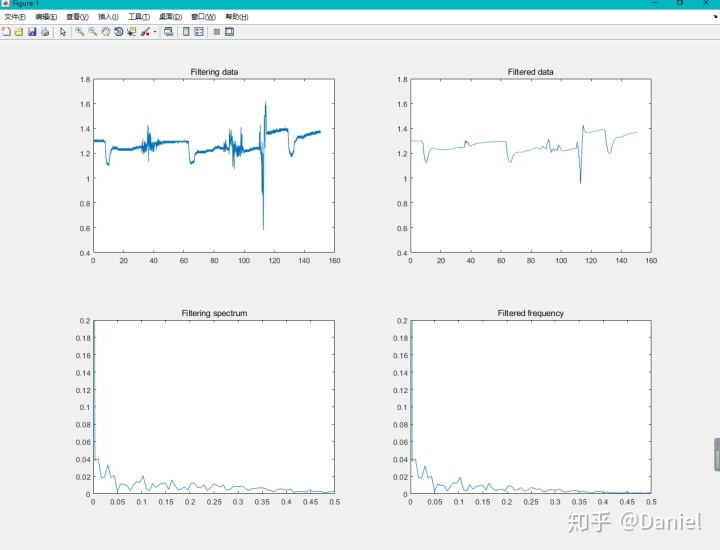截至频率 0.2Hz滤波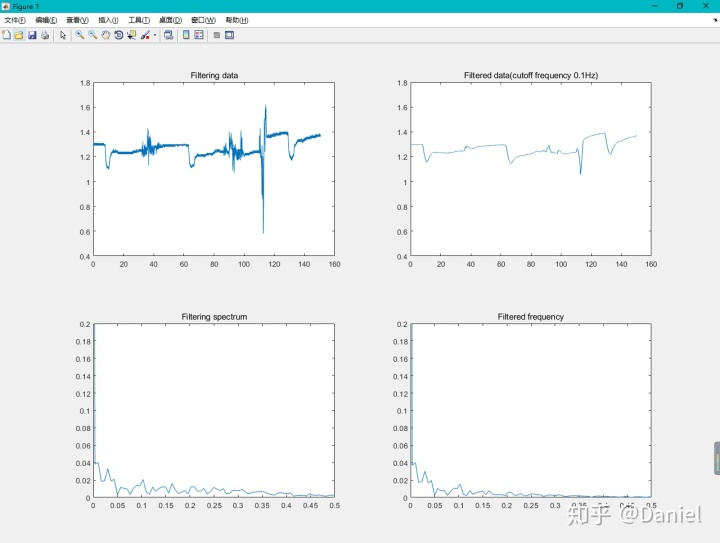截至频率 0.1Hz滤波

clc


展开全文• 222 2 2 2 基于 MATLAB 设计巴特沃斯低通滤波器 摘 要 首先分析了巴特沃斯低通滤波器的特性然后用MATLAB的信号处理工具箱提供的 函 数设计了巴特沃斯低通滤波器使得巴特沃斯滤波器的设计变得更加简单快捷直观 ...
• 本m文件基于matlab使用firpm函数计算出滤波器系数，从而完成了使用FIR滤波器拟合一阶RC电路的功能。这种思想可以扩展到其他的传输函数上。
• 姓名 XXX 学号 XX 专业 XXXXXX 课程设计题目 基于MATLAB设计巴特沃斯低通滤波器 指导教师 XX 主要内容: 本文重点研究怎样通过MATLAB来模拟出巴特沃斯低通滤波器讨论巴特沃斯低通滤波器在实际的一些应用提出了...
• 　%数字滤波器的指标 　wp=0.2*pi;ws=0.3*pi;Rp=1;As=40 　%转换成模拟域指标 　T=1;Fs=1/T; 　omegap=(2/T)*tan(wp/2); 　omegas=(2/T)*tan(ws/2); 　%模拟Butterworth滤波器的计算 　[cs,ds]=afd_buttap(omegap,...巴特沃斯
• 导出用于一阶 RC 低通滤波器的数字实现的传递函数，并绘制阶跃和频率响应。 详情请参考帖子： http://www.dsplog.com/2007/12/02/digital-implementation-of-rc-low-pass-filter/
• matlab实现FIR低通滤波器，并用它实现对噪声的滤除，每一步都加有注释，方便参考，程序运行无误
• ## 基于matlab设计的低通滤波器

千次阅读 多人点赞 2019-05-30 22:03:32
低通滤波器 %系统采样频率 fs=1200%原始信号 N=400; n=0:N-1; t=n/fs; fl=100 fh=400 y=sin(2*pi*fl*t)+sin(2*pi*fh*t);%显示原始信号 subplot(321) plot(t,y) title('输入信号');xlabel('t/s');ylabel('...
基于matlab设计的滤波器
低通滤波器
%系统采样频率
fs=1200%原始信号
N=400;
n=0:N-1;
t=n/fs;
fl=100
fh=400
y=sin(2*pi*fl*t)+sin(2*pi*fh*t);%显示原始信号
subplot(321)
plot(t,y)
title('输入信号');xlabel('t/s');ylabel('幅度');
%对原始信号频谱分析
sfft=fft(y);
subplot(322);
plot((1:length(sfft)/2)*fs/length(sfft),2*abs(sfft(1:length(sfft)/2))/length(sfft));
title('信号频谱');xlabel('频率/Hz');ylabel('幅度');
%设计低通滤波器
K = 2;
Wc =250;
[z,p,k] = buttap(K);        %z、p、k分别为零点列向量、极点列向量和增益系数
[b,a] = zp2tf(z,p,k);
[b,a] = lp2lp(b,a,2*pi*Wc);  % lp2lp转换命令把滤波器转化成具有不同截止频率的lp2hp,lp2bp和lp2bs
[b1,a1] = bilinear(b,a,fs);   %将模拟信号转为数字信号
[H,W] = freqz(b1,a1,N,fs);  %求数字低通滤波器的频率响应
subplot(323)
plot(W,abs(H));
title('低通滤波器');
grid on;
%滤波
y1=filter(b1,a1,y);          %叠加函数y经过低通滤波器以后的新函数
%显示滤波后的波形及频谱
subplot(324);
plot(t,y1);
title('输出信号');xlabel('t/s');ylabel('幅度');
y2=fft(y1);  %傅里叶变换
subplot(325);
plot((1:length(y2)/2)*fs/length(y2),2*abs(y2(1:length(y2)/2))/length(y2));
title('低通滤波后频谱');xlabel('频率/Hz');ylabel('幅度');  
展开全文• ## 用matlab做巴特沃斯低通滤波器

万次阅读 多人点赞 2020-07-11 23:00:39
matlab做巴特沃斯低通滤波器的干货 基本数据 fs=100 N=1024 n=-N/2:N/2-1 f=n*fs/N也可以表示为f=linspace(-fs/2,fs/2,N); t=n/fs 第一步：做出原信号的频谱函数 注意：用fft函数作频谱分析，得到的是0~fs内的频谱...
用matlab做巴特沃斯低通滤波器
趁着暑假，做一个心电图的matlab实验，遇到了滤波器问题，网上代码比较杂乱，做了一个汇总整理。 主要做了一个简单的低通滤波器并以三角函数为例子进行低通滤波。
基本数据
fk=100  %采样频率
N=1024  %采样个数
n=-N/2:N/2-1
f=n*fk/N
t=n/fk
y=sin(2*pi*10*t)

当然 f也可以表示成 f=linspace(-fk/2,fk/2,N);
第一步：做出原信号的频谱函数
注意：用fft函数作频谱分析，得到的是0~fk内的频谱 而用fftshift函数得到-fk/2~fk/2内的频谱
fft_y=fft(y);
fftshift_y=fftshift(fft_y);
f=linspace(-50,50,1024);
plot(f,abs(fftshift_y));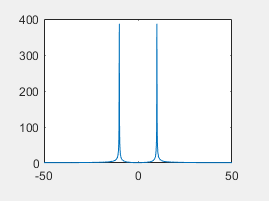第二步：做出巴特沃斯低通滤波器
用buttord函数求出阶数以及Wn
butter函数是求Butterworth数字滤波器的系数，在求出系数后对信号进行滤波时用filter函数 [g,Wn]=buttord(Wp,ws,Rp,Rs); Wp=fp/(0.5fk)=20/50=0.4(通带截至频率) Ws=fs/(0.5*fk)=30/50=0.6(阻带截止频率) Rp=1; 通带最大衰减 Rs=30; 阻带最小衰减 代码如下：
[g,Wn]=buttord(0.4,0.6,1,30);

用butter 求出差分方程的系数b a
不懂差分方程及filter用法的朋友可以点以下链接，参考这位大佬的理解 差分方程介绍
[b,a]=butter(g,Wn);

至此 准备工作都做完了 ，来看看这滤波器的相频特性啥样子
滤波器的相频特性
 [q,w]=freqz(b,a,256);
plot(w*fs/(2*pi),abs(q))

所以说 咱写出来的滤波器长这个样子 20Hz为通带截至频率 30Hz为阻带截至频率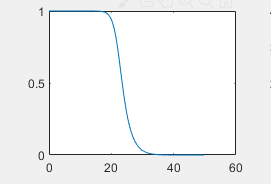第三步：用滤波器过滤信号 并得出频谱图
用filter过滤
用filter函数就能把原信号y过滤成k函数 ，需要注意的是，它们都是时域函数，需要进行fft变换才能看到频谱图
 k=filter(b,a,y);
fft_k=fft(k);
fftshift_k=fftshift(fft_k);
plot(f,abs(fftshift_k));

过滤后的结果如下：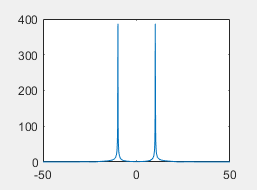没错，没变！我们低通滤波器允许20Hz以下的信号不变，而我们原信号的频率就是10Hz！
对高频的信号的低通滤波
这里就不赘述了，对y=sin(2pi40*t)的信号，依然用上面的低通过滤的结果如下图：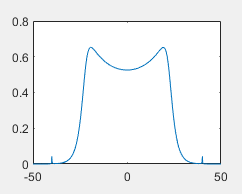不要看它长的怪，注意一下幅值，约等于0，即把40Hz的信号过滤掉了！ 行文至此，点个赞呗亲！
展开全文filter 过滤器 信号处理
• 低通滤波器 检查滤波器的性能，消除高频噪声，以平滑的信号。您将使用的模型包括一个模拟的、有噪声的信号和一个通过取移动平均值来选择低频的滤波器。 模拟信号为离散正弦波。“噪声”是通过叠加一个更高频率的...
低通滤波器
检查滤波器的性能，消除高频噪声，以平滑的信号。您将使用的模型包括一个模拟的、有噪声的信号和一个通过取移动平均值来选择低频的滤波器。
模拟信号为离散正弦波。“噪声”是通过叠加一个更高频率的正弦波而增加的。噪声信号通过一个滤波器，该滤波器将当前值与先前值取平均值。在离散系统一章中，您将学习更多关于如何构建这样的模型。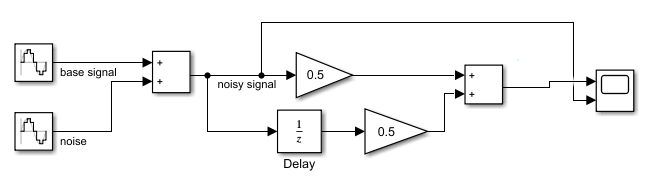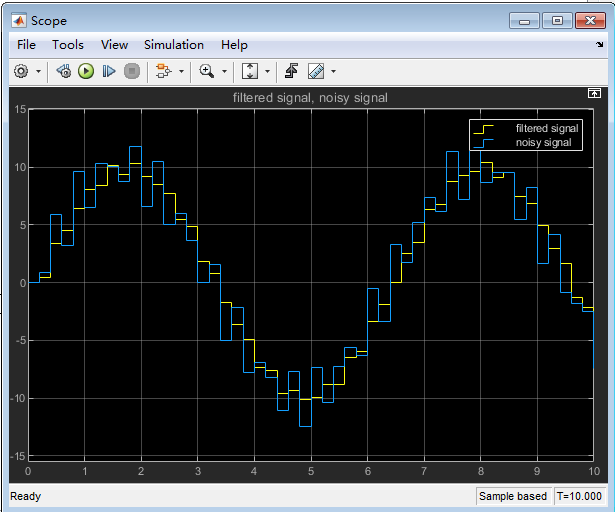展开全文• 精品文档 引言 当今数字信号处理技术正飞速发展它不但自成一门学科更是以不同的形式渗透到其他学 科它与国民经济息息相关与国防建设紧密相连它影响和改变着我们的生产生活方式因此 受到人们普遍的关注数字化智能化和......

# matlab中实现低通滤波器matlab 订阅